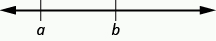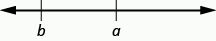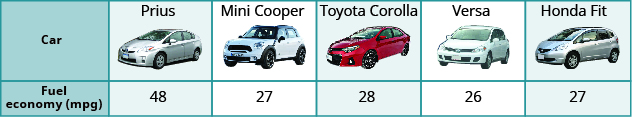Using Variables and Algebraic Notation

Learning Outcomes

• Use variables to represent unknown quantities in algebraic expressions
• Identify the variables and constants in an algebraic expression
• Use words and symbols to represent algebraic operations on variables and constants
• Use inequality symbols to compare two quantities
• Translate between words and inequality notation

Use Variables and Algebraic Symbols

Greg and Alex have the same birthday, but they were born in different years. This year Greg is $20$ years old and Alex is $23$, so Alex is $3$ years older than Greg. When Greg was $12$, Alex was $15$. When Greg is $35$, Alex will be $38$. No matter what Greg’s age is, Alex’s age will always be $3$ years more, right?

In the language of algebra, we say that Greg’s age and Alex’s age are variable and the three is a constant. The ages change, or vary, so age is a variable. The $3$ years between them always stays the same, so the age difference is the constant.

In algebra, letters of the alphabet are used to represent variables. Suppose we call Greg’s age $g$. Then we could use $g+3$ to represent Alex’s age. See the table below.

Greg’s age Alex’s age
$12$ $15$
$20$ $23$
$35$ $38$
$g$ $g+3$

Letters are used to represent variables. Letters often used for variables are $x,y,a,b,\text{ and }c$.

Variables and Constants

A variable is a letter that represents a number or quantity whose value may change.
A constant is a number whose value always stays the same.

To write algebraically, we need some symbols as well as numbers and variables. There are several types of symbols we will be using. In Whole Numbers, we introduced the symbols for the four basic arithmetic operations: addition, subtraction, multiplication, and division. We will summarize them here, along with words we use for the operations and the result.

Operation Notation Say: The result is…
Addition $a+b$ $a\text{ plus }b$ the sum of $a$ and $b$
Subtraction $a-b$ $a\text{ minus }b$ the difference of $a$ and $b$
Multiplication $a\cdot b,\left(a\right)\left(b\right),\left(a\right)b,a\left(b\right)$ $a\text{ times }b$ The product of $a$ and $b$
Division $a\div b,a/b,\frac{a}{b},b\overline{)a}$ $a$ divided by $b$ The quotient of $a$ and $b$

In algebra, the cross symbol, $\times$, is not used to show multiplication because that symbol may cause confusion. Does $3xy$ mean $3\times y$ (three times $y$ ) or $3\cdot x\cdot y$ (three times $x\text{ times }y$ )? To make it clear, use • or parentheses for multiplication.
We perform these operations on two numbers. When translating from symbolic form to words, or from words to symbolic form, pay attention to the words of or and to help you find the numbers.

• The sum of $5$ and $3$ means add $5$ plus $3$, which we write as $5+3$.
• The difference of $9$ and $2$ means subtract $9$ minus $2$, which we write as $9 - 2$.
• The product of $4$ and $8$ means multiply $4$ times $8$, which we can write as $4\cdot 8$.
• The quotient of $20$ and $5$ means divide $20$ by $5$, which we can write as $20\div 5$.

Exercises

Translate from algebra to words:

1. $12+14$
2. $\left(30\right)\left(5\right)$
3. $64\div 8$
4. $x-y$

Solution:

 1. $12+14$ $12$ plus $14$ the sum of twelve and fourteen
 2. $\left(30\right)\left(5\right)$ $30$ times $5$ the product of thirty and five
 3. $64\div 8$ $64$ divided by $8$ the quotient of sixty-four and eight
 4. $x-y$ $x$ minus $y$ the difference of $x$ and $y$

TRY IT

When two quantities have the same value, we say they are equal and connect them with an equal sign.

Equality Symbol

$a=b$ is read $a$ is equal to $b$
The symbol $=$ is called the equal sign.

An inequality is used in algebra to compare two quantities that may have different values. The number line can help you understand inequalities. Remember that on the number line the numbers get larger as they go from left to right. So if we know that $b$ is greater than $a$, it means that $b$ is to the right of $a$ on the number line. We use the symbols $\text{<}$ and $\text{>''}$ for inequalities.

$a<b$ is read $a$ is less than $b$
$a$ is to the left of $b$ on the number line$a>b$ is read $a$ is greater than $b$
$a$ is to the right of $b$ on the number lineThe expressions $a<b\text{ and }a>b$ can be read from left-to-right or right-to-left, though in English we usually read from left-to-right. In general,

$\begin{array}{l}a<b\text{ is equivalent to }b>a.\text{ For example, }7<11\text{ is equivalent to }11>7.\hfill \\ a>b\text{ is equivalent to }b<a.\text{ For example, }17>4\text{ is equivalent to }4<17.\hfill \end{array}$

When we write an inequality symbol with a line under it, such as $a\le b$, it means $a<b$ or $a=b$. We read this $a$ is less than or equal to $b$. Also, if we put a slash through an equal sign, $\ne$, it means not equal.

We summarize the symbols of equality and inequality in the table below.

Algebraic Notation Say
$a=b$ $a$ is equal to $b$
$a\ne b$ $a$ is not equal to $b$
$a<b$ $a$ is less than $b$
$a>b$ $a$ is greater than $b$
$a\le b$ $a$ is less than or equal to $b$
$a\ge b$ $a$ is greater than or equal to $b$

Symbols $<$ and $>$

The symbols $<$ and $>$ each have a smaller side and a larger side.

smaller side $<$ larger side

larger side $>$ smaller side

The smaller side of the symbol faces the smaller number and the larger faces the larger number.

Exercises

Translate from algebra to words:

1. $20\le 35$
2. $11\ne 15 - 3$
3. $9>10\div 2$
4. $x+2<10$

TRY IT

In the following video we show more examples of how to write inequalities as words.

Exercises

The information in the table below compares the fuel economy in miles-per-gallon (mpg) of several cars. Write the appropriate symbol $\text{=},\text{<},\text{ or }\text{>}$ in each expression to compare the fuel economy of the cars.

(credit: modification of work by Bernard Goldbach, Wikimedia Commons)1. MPG of Prius_____ MPG of Mini Cooper
2. MPG of Versa_____ MPG of Fit
3. MPG of Mini Cooper_____ MPG of Fit
4. MPG of Corolla_____ MPG of Versa
5. MPG of Corolla_____ MPG of Prius

TRY IT

Grouping symbols in algebra are much like the commas, colons, and other punctuation marks in written language. They indicate which expressions are to be kept together and separate from other expressions. The table below lists three of the most commonly used grouping symbols in algebra.

Common Grouping Symbols
parentheses $\left(\right)$
brackets $\left[\right]$
braces $\left\{\right\}$

Here are some examples of expressions that include grouping symbols. We will simplify expressions like these later in this section.

$\begin{array}{cc}8\left(14 - 8\right)21 - 3\\\left[2+4\left(9 - 8\right)\right]\\24\div \left\{13 - 2\left[1\left(6 - 5\right)+4\right]\right\}\end{array}$